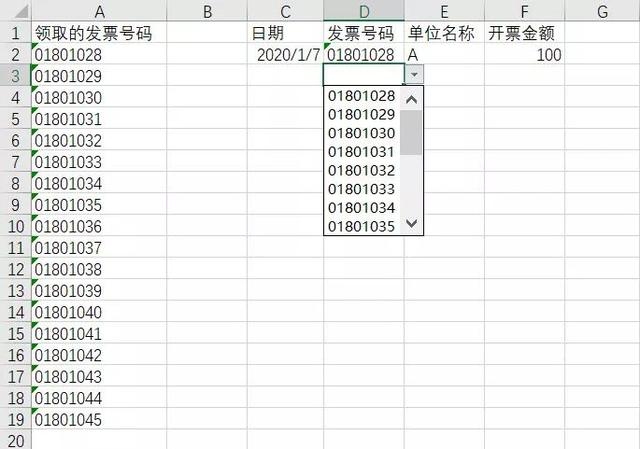﻿ 如何用vba在excel单元格中创建根据内容自动减少的数据有效性下拉列表？ - EXCEL VBA - ExcelOffice【微信公众号：水星Excel】

# 如何用vba在excel单元格中创建根据内容自动减少的数据有效性下拉列表？A列是一堆发票号码，D列的单元格中都做了下拉列表，数据来源自A列。

【01801028】。

```Private Sub Worksheet_SelectionChange(ByVal Target As Range)
Set oDic = CreateObject("Scripting.Dictionary")
If Target.Count = 1 And Target.Column = 4 Then
For i = 2 To Me.Range("a65536").End(xlUp).Row
'通过工作表函数判断是否已经存在，不存在则添加到字典中
If Excel.Application.WorksheetFunction.CountIf(Target.EntireColumn, Me.Cells(i, "a").Value) = 0 Then
End If
Next i
arr = oDic.keys
With Target
With .Validation
'先删除之前的数据有效性
.Delete
If UBound(arr) >= 0 Then
'再添加新的数据有效性下拉列表
End If
End With
End With
End If
End Sub```